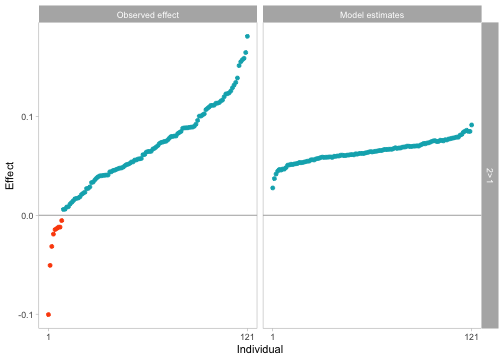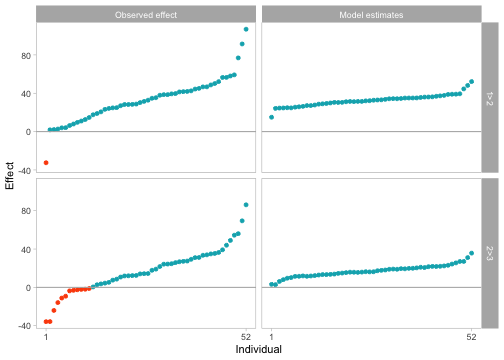# Quick Start

### Introduction

This is an R-package to assess qualitative individual differences using Bayesian model comparison and estimation.

quid uses Bayesian mixed models to estimate individual effect sizes and to test theoretical order constraints in repeated measures designs. It offers a method for testing the direction of individual effects. Typical questions that can be answered with this package are of the sort: “Does everyone show an effect in the same direction?” and “are there qualitative individual differences?”.

This is a quick start guide. For an extensive description of the package and the statistical models used see the main manual.

At this point the quid package can only be installed from github. For this you need to install the devtools package and then run the install_github function. You can include the argument build_vignettes = TRUE to also install this manual. This might take slightly longer to install. Lastly, you have to load the package via library:

devtools::install_github("lukasklima/quid", build_vignettes = TRUE)
library(quid)

### Overview of Functions

Function Description
constraintBF Main function to calculate Bayes factors for constraints
calculateDifferences Calculate differences between conditions specified in constraints
plotEffects Plot a BFBayesFactorConstraint object

### Impute Constraints

To impute constraints, use the whichConstraint argument of the constraintBF function. whichConstraint takes a named vector, where the names are the name of the effect factor and the values are the constraints on effect levels. For instance, say you have a column that holds the condition (condition) with levels treatment and control. You want to check if the outcome variable (outcome) is bigger in the treatment condition, so your input should look like this:

whichConstraint = c("condition" = "treatment > control")

If you have a third (or more) level(s), say a low dose treatment (low_dose) and you want to test whether the effect in the treatment condition is bigger than in the low dose condition and the effect in the low dose condition is bigger than in the control condition your input should look like this:

whichConstraint = c("condition" = "treatment > low_dose", "condition" = "low_dose > control")

In this example we use the stroop data set, which is part of quid. See ?stroop for details. We want to test whether the response time in seconds (rtS) is bigger in the incongruent (2) condition than in the congruent (1) condition.

#### Setting up the Analysis

We use a formula to express the model. The outcome variable rtS is modelled as a function of the main effect of ID (person variable), the main effect of cond (condition variable) and their interaction (ID:cond). In short, this can be expressed as ID*cond.

The whichRandom argument specifies that ID is a random factor. The ID argument specifies that the participants’ IDs are stored in the variable "ID". The rscaleEffects argument is used to specify priors for the fixed, random and interaction effect.

data(stroop)

resStroop <- constraintBF(formula = rtS ~ ID*cond,
data = stroop,
whichRandom = "ID",
ID = "ID",
whichConstraint = c("cond" = "2 > 1"),
rscaleEffects = c("ID" = 1, "cond" = 1/6, "ID:cond" = 1/10))

#### Interpreting the Output

Printing the resStroop object produces the following output:

resStroop
#>
#> Constraints analysis
#> --------------
#> Bayes factor          : 12.46582
#> Posterior probability : 0.6584444
#> Prior probability     : 0.05282
#>
#> Constraints defined:
#>  cond : 1 < 2
#>
#> =========================
#>
#> Bayes factor analysis
#> --------------
#>  ID                  : 3.945904e+428 ±0%
#>  cond                : 9.175351e+50  ±0%
#>  ID + cond           : 4.491989e+490 ±0.89%
#>  ID + cond + ID:cond : 1.341518e+491 ±1.57%
#>
#> Against denominator:
#>   Intercept only
#> ---
#> Bayes factor type: BFlinearModel, JZS

Under “Constraints analysis” you see the Bayes factor in favour of your defined constraints, where the full model  (under Bayes factor analysis) is in the denominator. So, the Bayes factor of the constraints analysis is the Bayes factor between the constrained model and model . Furthermore, you can see the posterior and prior probabilities of the constraints given the unconstrained model. You can think of the prior probability as the probability of the constraints holding before seeing the data, and the posterior probability as the probability of the constraints holding after seeing the data. We see that the constrained model is the preferred model.

Under “Bayes factor analysis” you can see the output from the generalTestBF function from the BayesFactor package. See the BayesFactor vignettes for details on how to manipulate Bayes factor objects. Model number three  with only main effects is the common effect model. Model number four  with the interaction term ID:cond allows for random slopes. The random effects model is the preferred model which suggests that the equality constraint does not hold. You can get a direct comparison between the two by manipulating the generalTestObj slot of the resStroop object:

bfs <- resStroop@generalTestObj
bfs / bfs
#> Bayes factor analysis
#> --------------
#>  ID + cond + ID:cond : 2.986467 ±1.81%
#>
#> Against denominator:
#>   rtS ~ ID + cond
#> ---
#> Bayes factor type: BFlinearModel, JZS

To get a comparison between the prefered model and all other models:

bfs / max(bfs)
#> Bayes factor analysis
#> --------------
#>  ID                  : 2.941373e-63  ±1.57%
#>  cond                : 6.839531e-441 ±1.57%
#>  ID + cond           : 0.3348438     ±1.81%
#>  ID + cond + ID:cond : 1             ±0%
#>
#> Against denominator:
#>   rtS ~ ID + cond + ID:cond
#> ---
#> Bayes factor type: BFlinearModel, JZS

#### Plotting Individual Effects

You can produce a plot of the individual effects; both the observed effects and the model estimates. The individual effects are the differences between the levels you specified in your constraints. In the plot below, we see the individual differences in response times between cond = 2 and cond = 1.

plotEffects(resStroop)We can see that the observed effects shows individuals with a negative effect. However, the model estimates are shrunk towards the grand mean and no individual has an estimated true negative effect.

If you want to manipulate the plot, you can do so by adding ggplot2 layers to it, or start from scratch by setting the .raw argument to TRUE to get the data.frame used to produce the plot.

plotEffects(resStroop, .raw = TRUE)

### Example ld5

We conclude this quick start guide by showing how to impute more than one constraint and how the output looks like. For this, we use the ld5 data set, which is part of quid. See ?ld5 for details.

The plotting function produces a comparison of observed effects versus the model estimates for each difference defined in your constraints. On the right side of the plot you see the labels of the differences.

data(ld5)

resLD5 <- constraintBF(formula = rt ~ sub * distance + side,
data = ld5,
whichRandom = c("sub"),
ID = "sub",
whichConstraint = c("distance" = "1 > 2", "distance" = "2 > 3"),
rscaleEffects = c("sub" = 1,
"side" = 1/6,
"distance" = 1/6,
"sub:distance" = 1/10))

plotEffects(resLD5)